# Python | Pandas Index.union()

• Last Updated : 22 Sep, 2021

Python is a great language for doing data analysis, primarily because of the fantastic ecosystem of data-centric python packages. Pandas is one of those packages and makes importing and analyzing data much easier.

Pandas Index.union() function form the union of two Index objects and sorts if possible. The function follows behaves like a standard set union operation. The function can also find the union of categorical data.

Syntax: Index.union(other)
Parameters :
other : Index or array-like
Returns : union : Index

Example #1: Use Index.union() function to find the union of two indexes.

## Python3

 `# importing pandas as pd``import` `pandas as pd` `# Creating the first index``idx1 ``=` `pd.Index([``10``, ``20``, ``18``, ``32``])` `# Creating the second index``idx2 ``=` `pd.Index([``21``, ``10``, ``30``, ``40``, ``50``])` `# Print the first Index``print``(idx1)` `# Print the second Index``print``(``"\n"``, idx2)`

Output :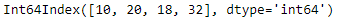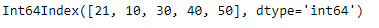Let’s find the union of these two indexes

## Python3

 `# perform set union of the two indexes``idx1.union(idx2)`

Output :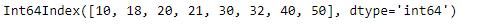The function has found the union of these two indexes.

Example #2: Use Index.union() function to perform set union operation on the given two indexes. Index labels are of string type.

## Python3

 `# importing pandas as pd``import` `pandas as pd` `# Creating the first index``idx1 ``=` `pd.Index([``'Harry'``, ``'Mike'``, ``'Arther'``, ``'Nick'``],``                                    ``name ``=``'Student'``)` `# Creating the second index``idx2 ``=` `pd.Index([``'Alice'``, ``'Bob'``, ``'Rachel'``, ``'Tyler'``, ``'Louis'``],``                                            ``name ``=``'Winners'``)` `# Print the first Index``print``(idx1)` `# Print the second Index``print``(``"\n"``, idx2)`

Output :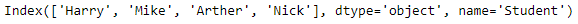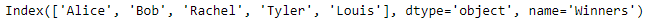Let’s find the union of these two indexes.

## Python3

 `# find union of two indexes``idx1.union(idx2)`

Output :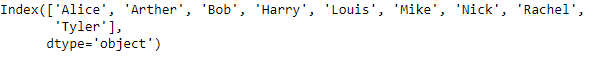The function has returned a new index that contains the result of the set union of the idx1 and idx2.

My Personal Notes arrow_drop_up